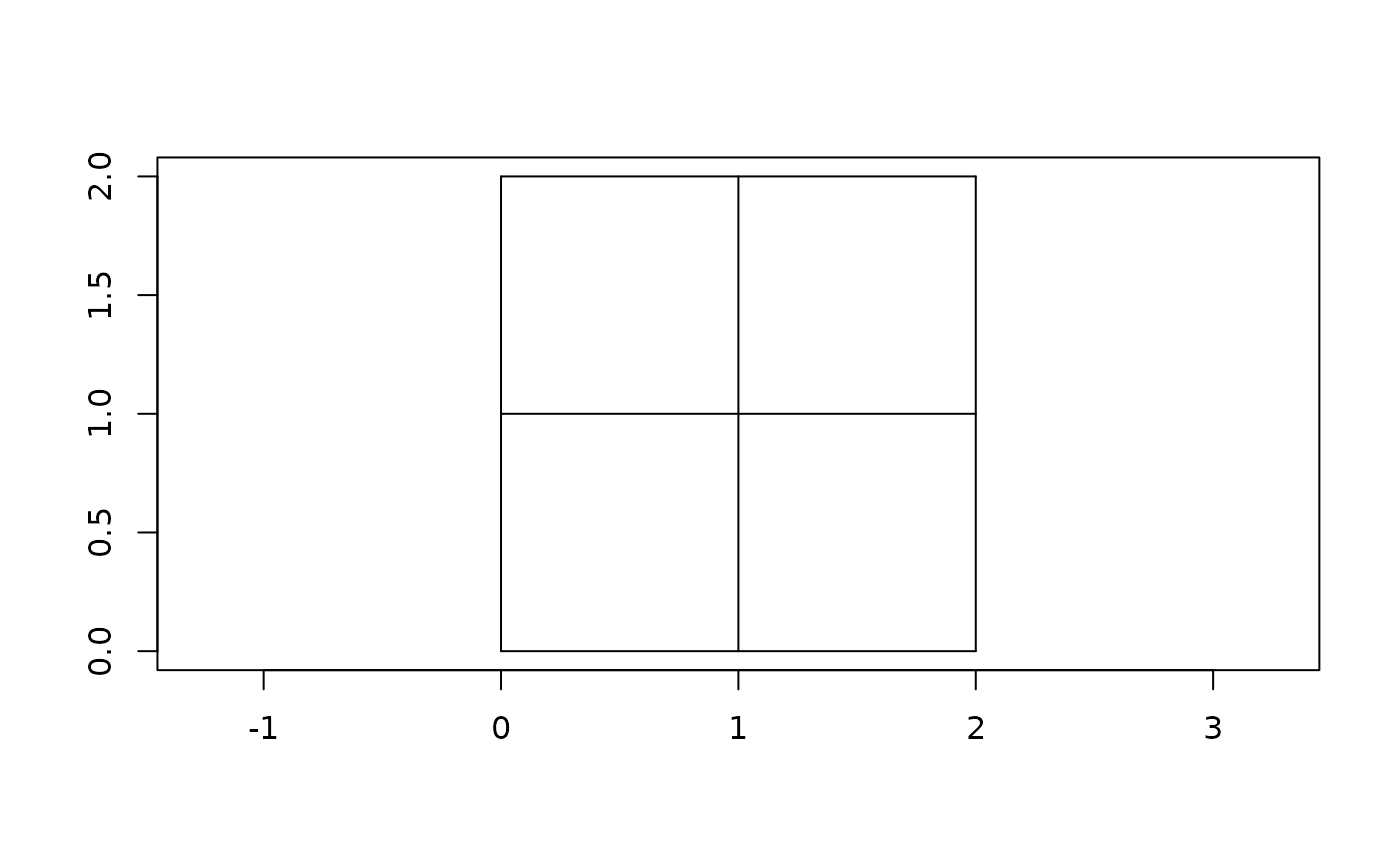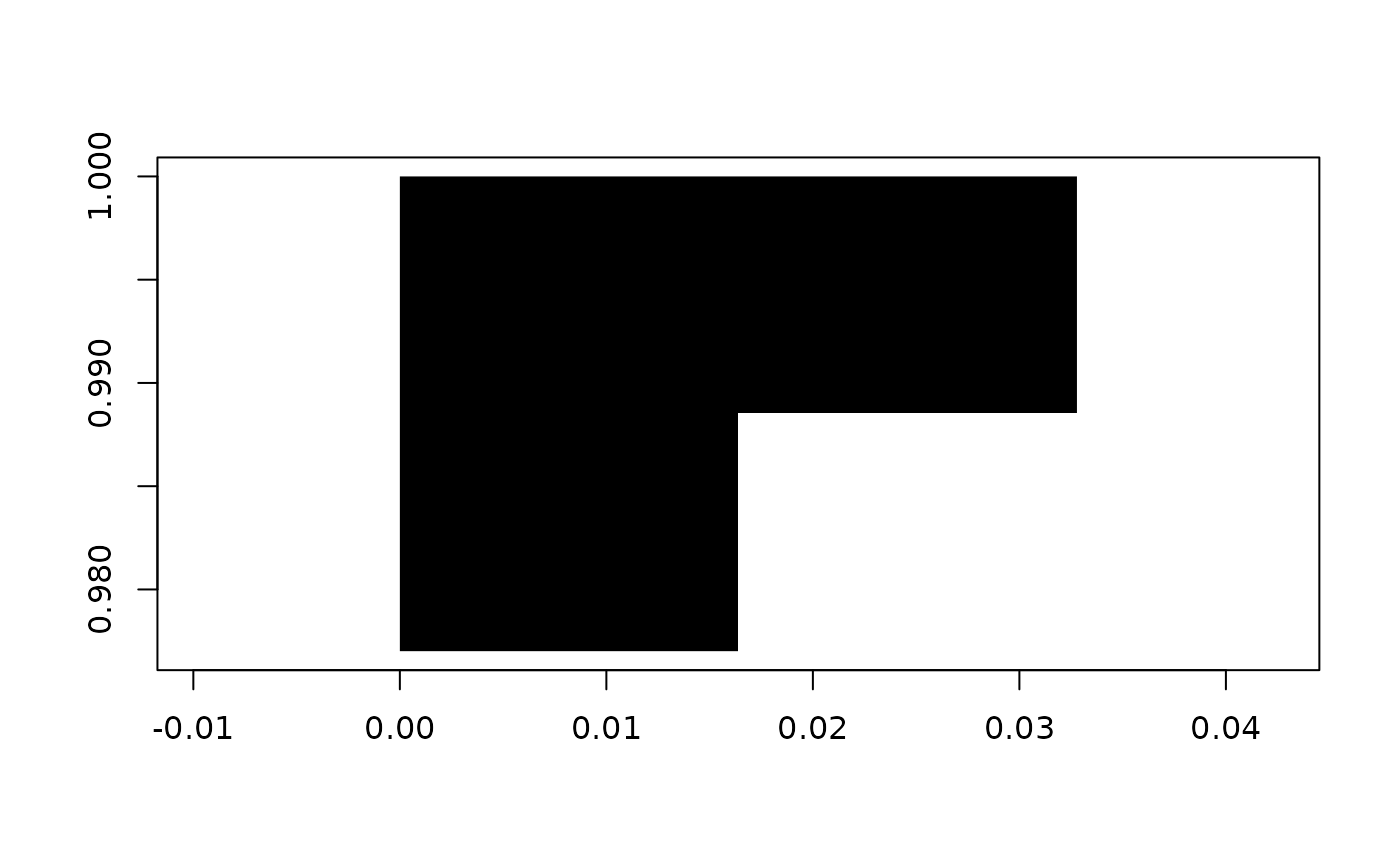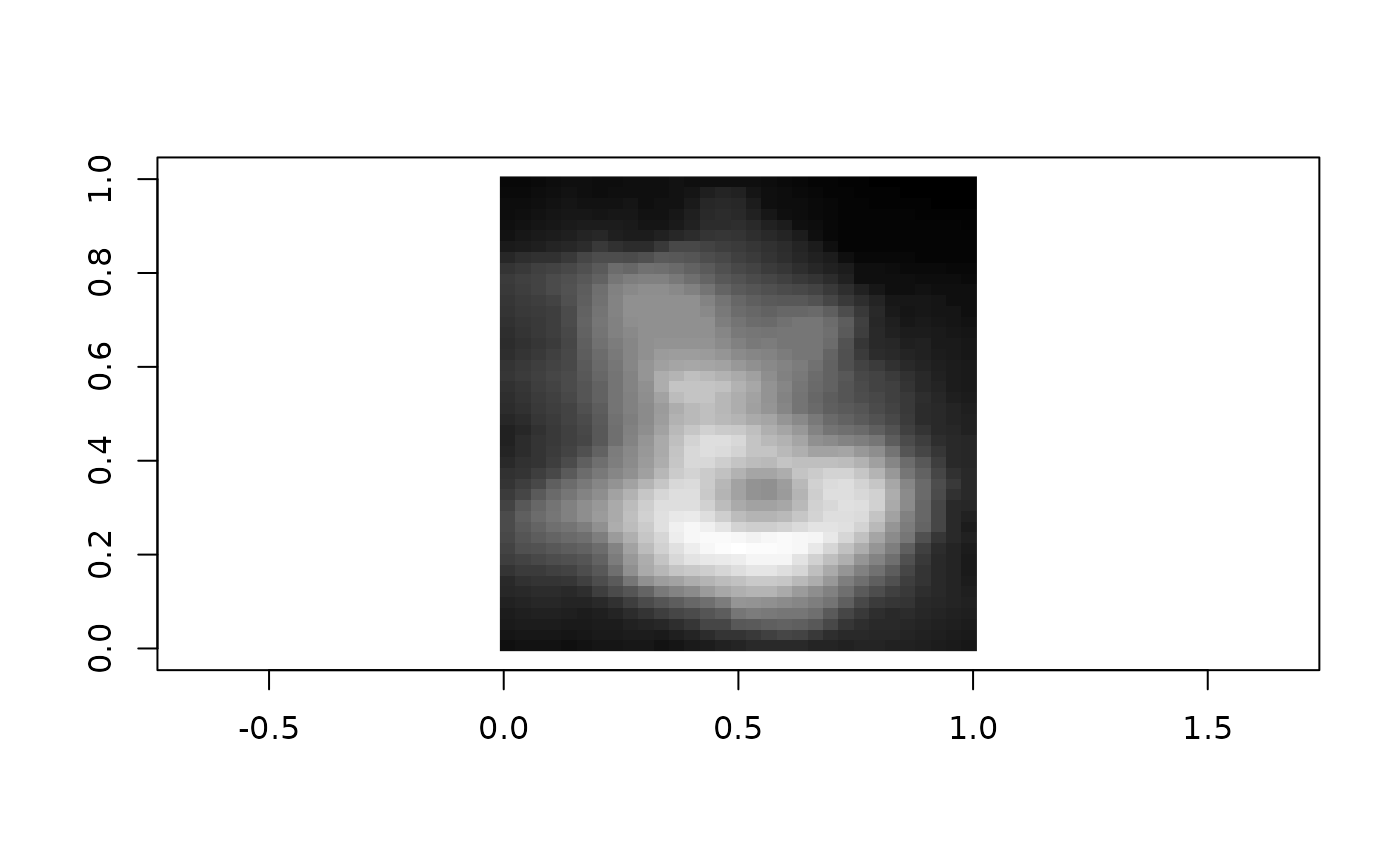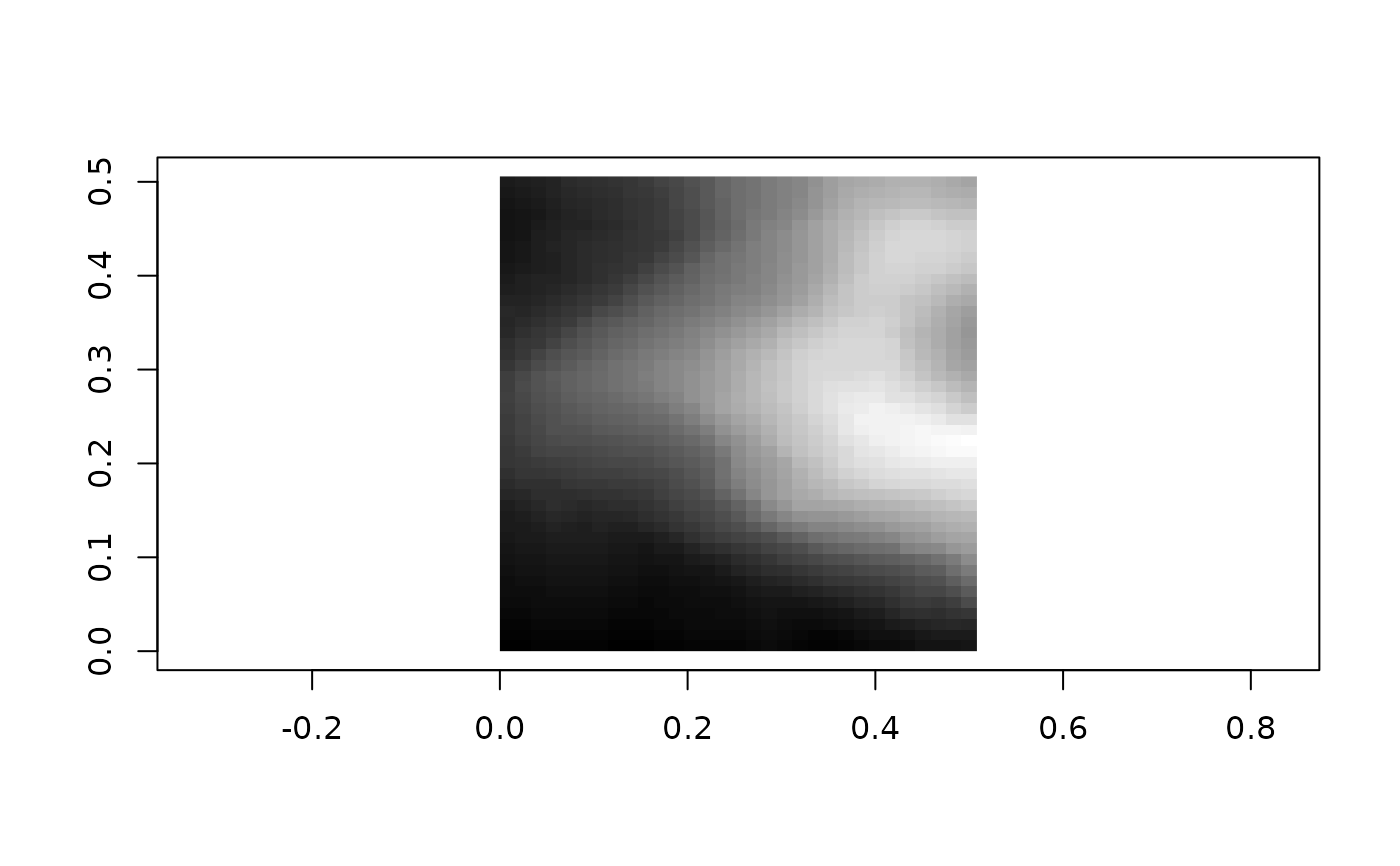grd() objects are just an array (any object with more than two dim()s) and a bounding box (a rct(), which may or may not have a wk_crs() attached). The ordering of the dimensions is y (indices increasing downwards), x (indices increasing to the right). This follows the ordering of as.raster()/rasterImage() and aligns with the printing of matrices.

grd(
bbox = NULL,
nx = NULL,
ny = NULL,
dx = NULL,
dy = NULL,
type = c("polygons", "corners", "centers")
)

grd_rct(data, bbox = rct(0, 0, dim(data), dim(data)))

grd_xy(data, bbox = rct(0, 0, dim(data) - 1, dim(data) - 1))

as_grd_rct(x, ...)

# S3 method for wk_grd_rct
as_grd_rct(x, ...)

# S3 method for wk_grd_xy
as_grd_rct(x, ...)

as_grd_xy(x, ...)

# S3 method for wk_grd_xy
as_grd_xy(x, ...)

# S3 method for wk_grd_rct
as_grd_xy(x, ...)

## Arguments

bbox

A rct() containing the bounds and CRS of the object. You can specify a rct() with xmin > xmax or ymin > ymax which will flip the underlying data and return an object with a normalized bounding box and data.

nx, ny, dx, dy

Either a number of cells in the x- and y- directions or delta in the x- and y-directions (in which case bbox must be specified).

type

Use "polygons" to return a grid whose objects can be represented using an rct(); use "centers" to return a grid whose objects are the center of the rct() grid; use "corners" to return a grid along the corners of bbox.

data

An object with two or more dimensions. Most usefully, a matrix.

x

An object to convert to a grid

...

Passed to S3 methods

## Value

• grd() returns a grd_rct() for type == "polygons or a grd_xy() otherwise.

• grd_rct() returns an object of class "wk_grd_rct".

• grd_xy() returns an object of class "wk_grd_xy".

## Examples

# create a grid with no data (just for coordinates)
(grid <- grd(nx = 2, ny = 2))
#> <wk_grd_rct [2 x 2 x 0] => [0 0 2 2]>
#> List of 2
#>  $data: logi[1:2, 1:2, 0 ] #>$ bbox: wk_rct[1:1] [0 0 2 2]
#>  - attr(*, "class")= chr [1:2] "wk_grd_rct" "wk_grd"
as_rct(grid)
#> <wk_rct>
#>  [0 1 1 2] [0 0 1 1] [1 1 2 2] [1 0 2 1]
as_xy(grid)
#> <wk_xy>
#>  (0.5 1.5) (0.5 0.5) (1.5 1.5) (1.5 0.5)
plot(grid, border = "black")# more usefully, wraps a matrix or nd array + bbox
# approx volcano in New Zealand Transverse Mercator
bbox <- rct(
5917000,       1757000 + 870,
5917000 + 610, 1757000,
crs = "EPSG:2193"
)
(grid <- grd_rct(volcano, bbox))
#> <wk_grd_rct [87 x 61] => [5917000 1757000 5917610 1757870] with crs=EPSG:2193>
#> List of 2
#>  $data: num [1:87, 1:61] 97 97 98 98 99 99 99 99 100 100 ... #>$ bbox: wk_rct[1:1] [5917000 1757000 5917610 1757870]
#>  - attr(*, "class")= chr [1:2] "wk_grd_rct" "wk_grd"

# these come with a reasonable default plot method for matrix data
plot(grid)# you can set the data or the bounding box after creation
grid\$bbox <- rct(0, 0, 1, 1)

# subset by indices or rct
plot(grid[1:2, 1:2])plot(grid[c(start = NA, stop = NA, step = 2), c(start = NA, stop = NA, step = 2)])plot(grid[rct(0, 0, 0.5, 0.5)])